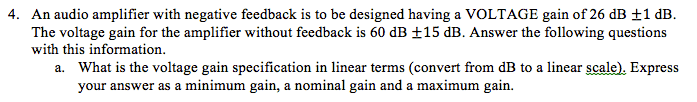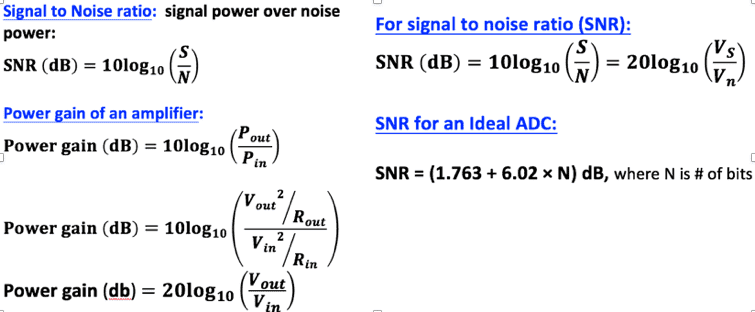# Audio Amp with negative feedback

## Homework Statement## Homework Equations## The Attempt at a Solution

I am not really sure how to do this problem. How can I convert from db to linear terms just given db? Don't I need more information? For do I have to do a system of equations or something using the Power gain = 20log(vo/vi)? I'm not even sure if these are the right equations to use.

You aren't missing any problem information but you might be missing concepts. We can convert ratios to there corresponding db this way.
$$\text{any ratio of quantity Q in (db)} = 10 \log_{10} \left(\frac{Q_1}{Q_2}\right)$$
This tells us
$$\text{Voltage Gain (db)} = 10 \log_{10} \frac{V_{out}}{V_{in}}$$
##\text{Power} = V^2/R## connects the concepts of voltage and power gain. For this, ##R_{out} = R_{in}## which explains the bottom left formula you posted (a long with a log property).
$$\text{Power Gain (db)} = 20 \log_{10} \frac{V_{out}}{V_{in}}$$
This should be enough to relate different gains and obtain the linear voltage gain ## \frac{V_{out}}{V_{in}}##

•Tom.G and Merlin3189
rude man
Homework Helper
Gold Member
Voltage gain is by convention 20 log10(Vout/Vin).

Last edited:
Voltage gain is by convention 20 log1010(Vout/Vin).
That's the formula supplied for power gain by the OP. I don't think they are the same.

•Tom.G
rude man
Homework Helper
Gold Member
That's the formula supplied for power gain by the OP. I don't think they are the same.
It is if input and output resistances are the same (e.g. 50 ohms is typical for high-freq equipmt.)
But otherwise, no. Power is 10 log10(Pout/Pin).

Tom.G
Gold Member
20 log10(Vout/Vin).

Power is 10 log10(Pout/Pin)

They are both correct for Power Gain when impedances are the same for input and output.
10 V, 50 Ohms = 100/50 = 2W (P1)
3.16V, 50 Ohms = 10/50 = 0.2W (P2)

10*Log10(P1/P2) ; == ; 10*Log10(2/0.2) = 10dB
20*Log10(V1/V2) ; == ; 20*Log10(10/3.16) = 10dB

This is because in the first case you are calculating power gain using ratios of powers, all the same units.
In the second case, you are calculating power gain using ratios of voltages. Mixed units. Power being proportional to the square of voltage, and we aren't squaring the voltage as part of the ratio, we instead multiply the log by 2; effectively squaring the ratio.

Following the above verbiage, the voltage gain would be:
10*Log10(V1/V2) ; == ; 10*Log10(10/3.16) = 5dB

rude man
Homework Helper
Gold Member
Following the above verbiage, the voltage gain would be:
10*Log10(V1/V2) ; == ; 10*Log10(10/3.16) = 5dB
Well I wouldn't follow that verbiage!
Voltage gain = 20 log(10/3.16) = 10 dB.

Tom.G
Well, after more research than it should have taken, I agree with you.